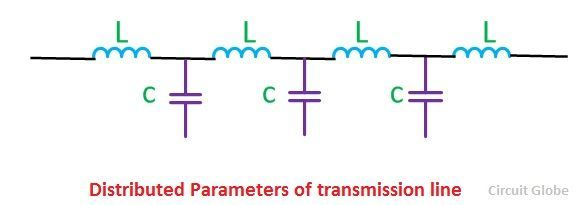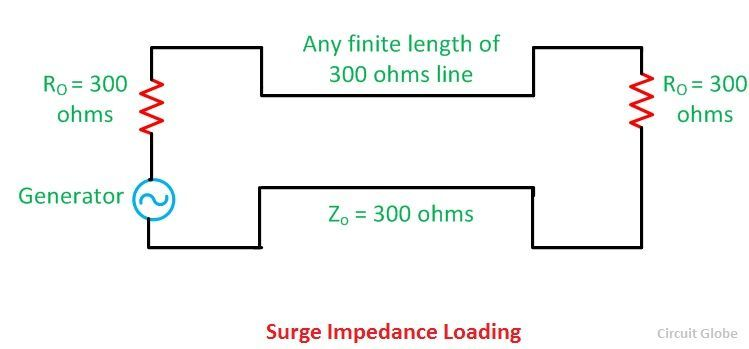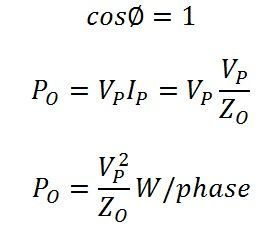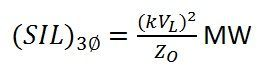Capacitance and reactance are the main parameters of the transmission line. It is distributed uniformly along the line. These parameters are also called distributed parameters. When the voltage drops occur in transmission line due to inductance, it is compensated by the capacitance of the transmission line.The transmission line generates capacitive reactive volt-amperes in its shunt capacitance and absorbing reactive volt-amperes in its series inductance.The load at which the inductive and capacitive reactive volt-amperes are equal and opposite, such load is called surge impedance load.

It is also called natural load of the transmission line because power is not dissipated in transmission. In surge impedance loading, the voltage and current are in the same phase at all the point of the line. When the surge impedance of the line has terminated the power delivered by it is called surge impedance loading.

Shunt capacitance charges the transmission line when the circuit breaker at the sending end of the line is close. As shown belowLet V = phase voltage at the receiving end
L = series inductance per phase
XL = series inductance reactance per phase
XC = shunt capacitance reactance per phase

Capacitive volt-amperes (VAr) generated in the lineThe series inductance of the line consumes the electrical energy when the sending and receiving end terminals are closed.Inductive reactive volt-amperes (VAr) absorbed by the lineUnder natural load, the reactive power becomes terminated, and the load becomes purely resistive.And it is calculated by the formula given belowSurge impedance loading is also defined as the power load in which the total reactive power of the lines becomes zero. The reactive power generated by the shunt capacitance is consumed by the series inductance of the line.

If Po is its natural load of the lines, (SIL)1∅ of the line per phaseSince the load is purely resistive,Thus, per phase power transmitted under surge impedance loading is (VP2)/ZO watts, Where Vp is the phase voltage.If kVL is the receiving end voltage in kV, thenSurge impedance loading depends on the voltage of the transmission line. Practically surge impedance loading always less than the maximum loading capacity of the line.

If the load is less than the SIL, reactive volt-amperes are generated, and the voltage at the receiving end is greater than the sending end voltage. On the other hand, if the SIL is greater than the load, the voltage at receiving end is smaller because the line absorbs reactive power.

If the shunt conductance and resistance are neglected and SIL is equal to the load than the voltage at both the ends will be equal.

### Conclusion

Surge impedance load is the ideal load because the current and voltage are uniform along the line. The wave of current and voltage is also in phase because the reactive power consumed are equal to the reactive power generated by the transmission line.